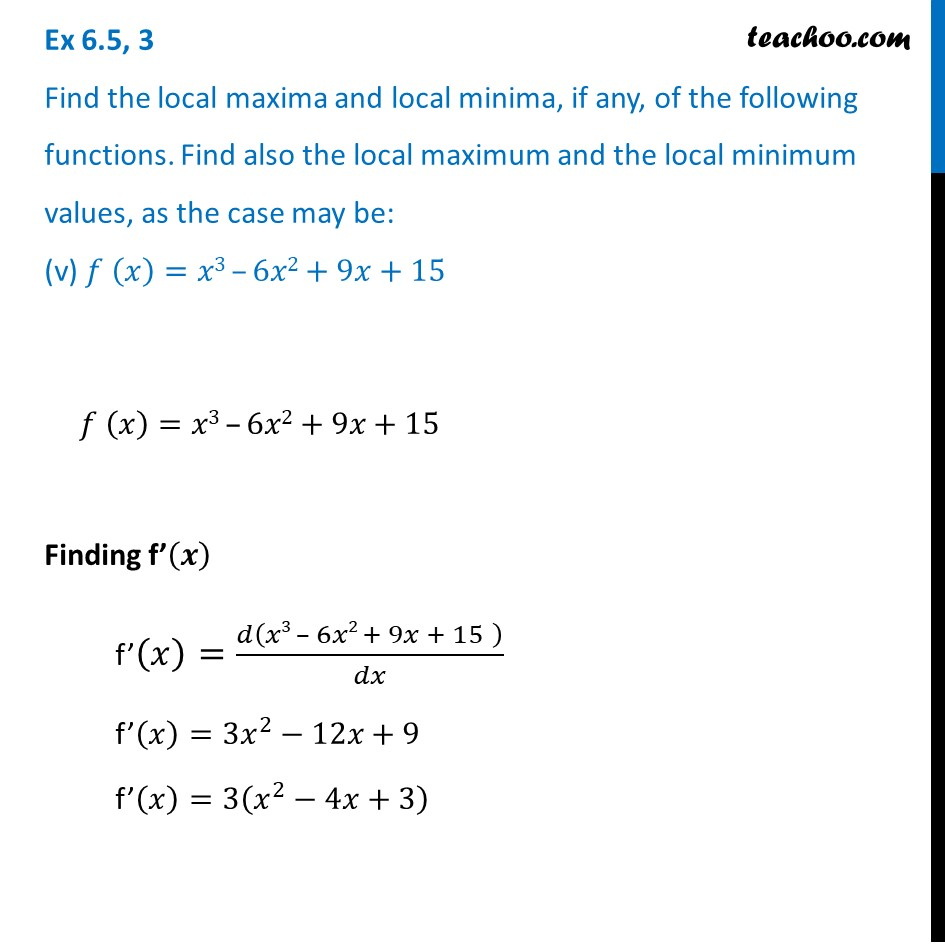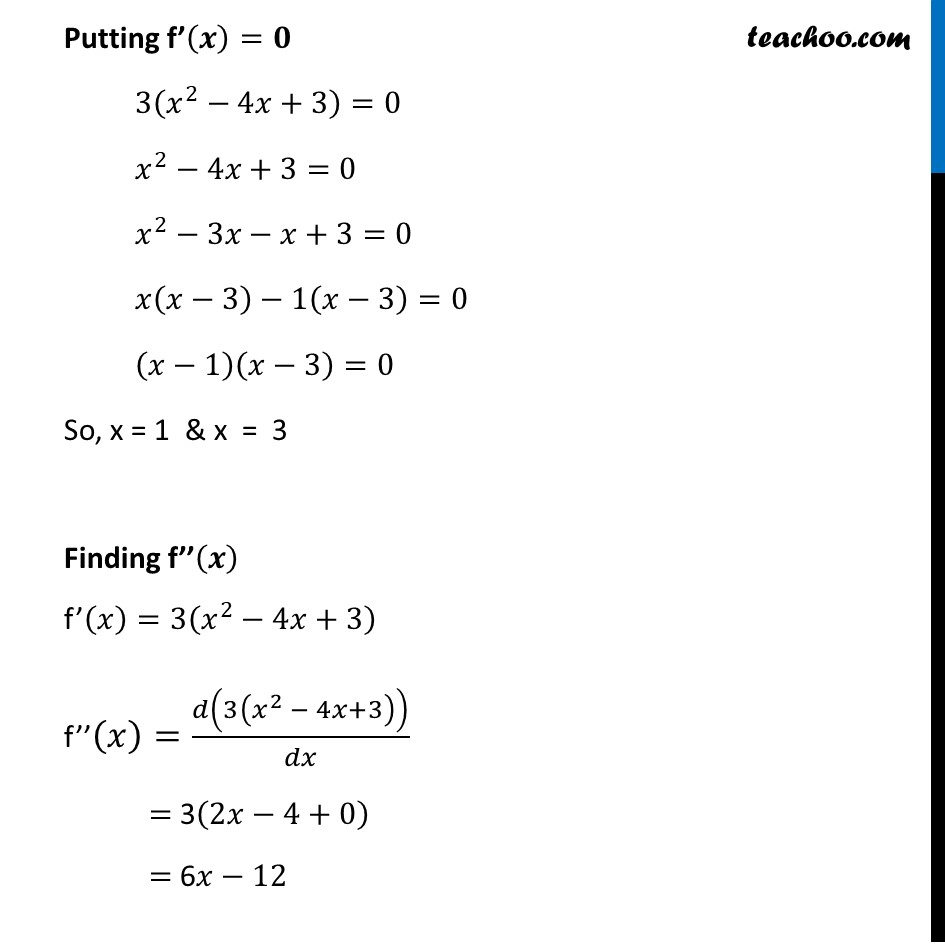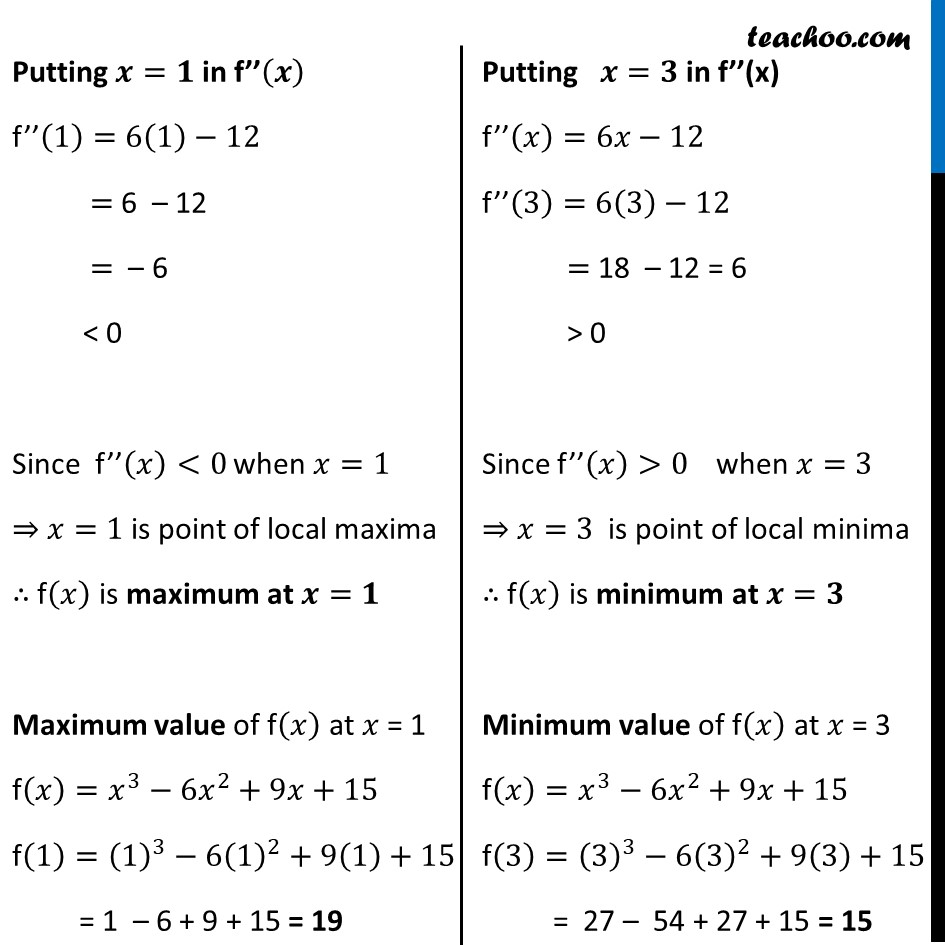Ex 6.5

Chapter 6 Class 12 Application of Derivatives
Serial order wiseMaths Crash Course - Live lectures + all videos + Real time Doubt solving!

### Transcript

Ex 6.5, 3 Find the local maxima and local minima, if any, of the following functions. Find also the local maximum and the local minimum values, as the case may be: (v) 𝑓 (𝑥)=𝑥3 –6𝑥2+9𝑥+15𝑓 (𝑥)=𝑥3 –6𝑥2+9𝑥+15 Finding f’(𝒙) f’(𝑥)=𝑑(𝑥3 – 6𝑥2 + 9𝑥 + 15" " )/𝑑𝑥 f’(𝑥)=3𝑥^2−12𝑥+9 f’(𝑥)=3(𝑥^2−4𝑥+3) Putting f’(𝒙)=𝟎 3(𝑥^2−4𝑥+3)=0 𝑥^2−4𝑥+3=0 𝑥^2−3𝑥−𝑥+3=0 𝑥(𝑥−3)−1(𝑥−3)=0 (𝑥−1)(𝑥−3)=0 So, x = 1 & x = 3 Finding f’’(𝒙) f’(𝑥)=3(𝑥^2−4𝑥+3) f’’(𝑥)=𝑑(3(𝑥^2 − 4𝑥+3))/𝑑𝑥 = 3(2𝑥−4+0) = 6𝑥−12 Putting 𝒙=𝟏 in f’’(𝒙) f’’(1)=6(1)−12 = 6 – 12 = – 6 < 0 Since f’’(𝑥)<0 when 𝑥=1 ⇒ 𝑥=1 is point of local maxima ∴ f(𝑥) is maximum at 𝒙=𝟏 Maximum value of f(𝑥) at 𝑥 = 1 f(𝑥)=𝑥^3−6𝑥^2+9𝑥+15 f(1)=(1)^3−6(1)^2+9(1)+15 = 1 – 6 + 9 + 15 = 19 Putting 𝒙=𝟑 in f’’(x) f’’(𝑥)=6𝑥−12 f’’(3)=6(3)−12 = 18 – 12 = 6 > 0 Since f’’(𝑥)>0 when 𝑥=3 ⇒ 𝑥=3 is point of local minima ∴ f(𝑥) is minimum at 𝒙=𝟑 Minimum value of f(𝑥) at 𝑥 = 3 f(𝑥)=𝑥^3−6𝑥^2+9𝑥+15 f(3)=(3)^3−6(3)^2+9(3)+15 = 27 – 54 + 27 + 15 = 15